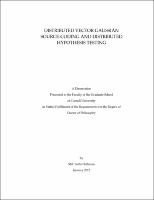## Distributed Vector Gaussian Source-Coding And Distributed Hypothesis Testing##### Abstract
Distributed compression is desired in applications in which data is collected in a distributed manner by several sensors and information about the data is sent to a processing center, which uses these information to meet an end goal. In this work, we focus on two such applications: (1) distributed source coding and (2) distributed hypothesis testing. In distributed source coding, we determine the rate region of the vector Gaussian one-helper source-coding problem under a covariance matrix distortion constraint. The rate region is achieved by a simple scheme that separates the lossy vector quantization from the lossless spatial compression. We introduce a novel analysis technique, namely distortion projection. The converse is established by combining distortion projection with two other analysis techniques that have been employed in the past to obtain partial results for the problem. We also study an extension to a special case of the problem in which the primary source is a vector and the helper's observation is a scalar and consider separate distortion constraints on both sources. We provide an outer bound to the rate region of this problem and show that it is partially tight in general and completely tight in some nontrivial cases. In distributed hypothesis testing, we study a problem in which data is compressed distributively and sent to a detector that seeks to decide between two possible distributions for the data. The aim is to characterize all achievable en- coding rates and exponents of the type 2 error probability when the type 1 error probability is at most a fixed value. For related problems in distributed source coding, schemes based on random binning perform well and are often optimal. For distributed hypothesis testing, however, the use of binning is hindered by the fact that the overall error probability may be dominated by errors in the binning process. We show that despite this complication, binning is optimal for a class of problems in which the goal is to "test against conditional independence." We then use this optimality result to give an outer bound for a more general class of instances of the problem. We also extend the "test against independence" result of Ahlswede and Csisz´ r to the vector Gaussian case. a
2012-01-31
##### Keywords
Source coding; Vector Gaussian source; One-helper problem; Matrix Constraint; Distributed hypothesis testing; Binning
Wagner, Aaron B.